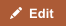Kilometer and Mile are both units of distance. In the United States and the United Kingdom, distances are measured in miles. In most other countries, the metric system is used and distances are measured in kilometers.

Comparison chart

Kilometer versus Mile comparison chartKilometerMile
Yards 1 km = 1093.613 yd 1 mile = 1,760 yd
Feet 1 km = 3280.84 ft 1 mile = 5,280 ft
Meters 1 km = 1000 m 1 mile = 1609.344 m
Inches 1 km = 39,370 in 1 mile = 63,360 in
Kilometers 1 km = 1 km 1 mile = 1.609 km
Miles 1 km = 0.621 mi 1 mile = 1 mile
Centimeters 1 km = 100000 cm 1 mile = 160934.4 cm

Mile to Kilometer conversion

• 1 mile = 1.609 kilometers
• 1 mile = 5,280 feet
• 1 mile = 1,760 yards

Kilometer to Mile Conversion

• 1 kilometer = 0.621 miles
• 1 kilometer = 3,280.8 feet
• 1 kilometer = 1,093.6 yards
• 1 kilometer = 1,000 m

Trivia

• Kilometer is the American spelling for Kilometre.
• A nautical mile is 1.85 kilometers